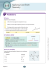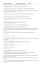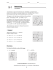# 3 transformations

## Transcription

3 transformations
```Transformations
Dilations
Translations
Reflections
Rotations
Reflections in a line
• Reflections in a line are like mirror images.
• They stay the same size and shape, but they flip!
• In geometry, reflections of figures may be on
a coordinate plane.
Reflections in a line
• Reflections can be made across the x-axis.
x-axis
Look at the corresponding points in the figures.
The point (-4, 4) corresponds to the image point (-4, -4).
The point (2, 4) corresponds to (2, -4).
Notice that in a reflection over the x-axis, the coordinates of
the x’s stay the same but the y’s change sign.
In a reflection across the x-axis, the point (x, y)
reflects onto image (x, -y).
Reflections in the y axis
• Reflections can be made across the y-axis.
y-axis
Check the corresponding points here.
Notice that the point (2, 1) corresponds to (-2, 1).
The point (7, 1) corresponds to (-7, 1).
The y values stay the same, but the x values change sign.
In a reflection across the y axis,
the point (x, y) reflects onto image (-x, y).
Reflections in other lines . . .
• Let’s look at what happens if you reflect a figure across the
line y = x
or
line y = -x
y=x
Look at corresponding points.
Notice that for (x, y), the
corresponding image point is
(y, x). For (-2, 5), image point
is (5, -2).
y = -x
Look at corresponding points.
Notice that for (x, y), the
corresponding image point is
(-y, -x). For (6, 3), image point
is (-3, -6)
Reflections in more lines . . .
• What happens if you reflect in a line
y = 3?
or
x = -2 ?
y=3
x = -2
Each point and corresponding image
must be equidistant from the line.
Note (4, 2) and image point (4, 4)
are each 1 unit from the line y = 3.
Each point and corresponding image
must be equidistant from the line.
Note (0, 4) and image point (-4, 4)
are 2 units from the line x = -2.
Check it out !
See how you can do with these problems.
Find the image point for each reflection given the point and the
line of reflection:
a. Point (5, -2), reflected in the x axis
b. Point (- 4, 3), reflected in the line y = x
c. Point (-1, -6), reflected in the y axis
d. Point (2, 4), reflected in the line y = 1
e. Point (-3, 5), reflected in the line x = 3
f. Point (4, 7) reflected in the y axis
b. (3, - 4)
c. (1, - 6)
d. (2, -2) e. ( 9, 5)
f. (-4, 7)
Translations (or slides)
• Figures which are transformed by “sliding”
from one place to another without change to
size, shape, or direction illustrate “translation”.
Notice all the faces are the same. They just move
around the page!
Let’s move some things!
• When we slide or translate figures,
nothing changes except the location.
• In the figure to the right, triangle A
slides 7 units to the right and 4 units
down to the image position A’. Each
vertex of the triangle makes that
move.
AA’
We can explain the translation in several ways.
1. Describe it in words: “ Move the figure 7 units right and 4 units down.”
2. Use symbols: Using T (for transition) and a coordinate pair to represent
the right/left move and then the up/down move , write T(7, -4).
3. Show a mapping: (x, y ) (x + 7, y – 4)
Check it out . . . .
1.
Under translation T(-3, 2), what will the point (4, 5) become?
2.
Under translation (x, y) (x+1, y-4), what will (2, 5) become?
3.
Under the translation of 4 units up and 3 unit left, what will (5, -1) become?
4.
Rectangle ABCD with vertices shown is translated under T(-2, 1). Find the
vertices of rectangle A’B’C’D’.
A(1, 7)  A’( , ) C(1, 2)  C’( , )
B(6, 7)  B’ ( , )
D(6, 2)  D’( , )
5.
ΔABC with vertices shown below is translated to ΔA’B’C’.
A(4, 7), B (1, 0), C ( , )  A’(1, 5), B’ ( , ), C’(4, 0)
a. What translation is being used here?
b. Find the coordinates of point C.
c. Find the coordinates of point B’.
(continued on next slide)
Keep it going . . . .
B
a.
A
A’
c
.
C
b.
A
D
7.
6. a. Is this a translation?
b. Is this a translation?
c. Is this a translation?
a. What point is the image of
B under T(7, -10)?
b. What point is the image of
D under T (-5, 4)?
c. What point is the image of
C under T(7, 4)?
8. What translation is
used in the figures
above?
9. If point A(-3, 1) is translated under T(2, -3), followed by T(5, 1), followed by T
(-1, 6), what single translation would have given the same result?
5.
a. T(-3, -2),
7. a. D,
b. C,
2. (3, 1)
b. C(7, 2),
c. A
3. (2, 3)
c. B’(-2, -2)
A8. T(6, 3)
4. A’(-1, 8), B’(4, 8), C’(-1, 3), D’(4, 3)
6. a. no.
b. yes
c. no
9. T (6, 4)
Dilations
• A dilation is a non-rigid transformation that results in
an image that is the same shape as the original but is
either smaller or larger. Every image is similar to its
pre-image.
With dilations, the corresponding angles stay the same.
9
12
A
15
3
4
A’
5
• Corresponding linear measures (length, width, radius, etc.)
are proportional. Their ratios all reduce to the same value.
• The corresponding sides above create the following ratios:
5 4
3
1
, , and which all reduce to .
15 12
9
3
Since all sides of the first triangle can be multiplied by 1/3 (or
divided by 3) to get the measures of the second triangle, the value
1/3 is called a scale factor of the dilation.
Scale factors and centers . . .
• Dilations use a scale factor to describe the size change from
the original to the image. It represents the ratio of the lengths
of the sides of the two figures.
• A center is also part of the dilation process. In coordinate
geometry, this is usually, but not always, the origin (0, 0).
•Here’s a sample dilation where the
smaller green rectangle has a
dilation image, the red rectangle,
with center at (0, 0), and a scale
factor of 2.
A(-2, 1)
B(2, 1)
D’
•Notice that all of the coordinates of
the red rectangle are 2 times those of
the green rectangle.
B’
A’
A
B
D
C
C(2 -1)
D(-2,-1)
A’(-4. 2)
C’
B’(4, 2)
C’(4, -2)
D’(-4, -2)
.
Dilations and notation . . .
• A dilation with scale factor “k” and center at (0,0) may be
expressed as Dk(x,y) = (kx, ky).
If the scale factor is between 0 and 1, the image will be smaller than
the original, or a reduction.
Scale factor = 3/4
If the scale factor is greater than 1, the image will be larger than the
original, or an enlargement.
Scale factor = 2
A dilation with center at (0, 0) and a
scale factor of 2/3. . .
• In this dilation, notice the
center is (0, 0) and the sides
of the image in red are 2/3
the lengths of the original
figure in blue.
• Since the scale factor is less
than 1, the image (red) is a
reduction of the original
figure (blue).
Dilations with center
not at (0, 0)
• If the center is not at (0, 0), be careful to check
the lengths of the sides of the figures to
conform to the scale factor.
A
The center of the dilation is at
point A. The original figure is
the red rectangle, and the image
is the green rectangle. The scale
factor must be 2 since each of the
sides of the image (green) is 2
times the length of the sides of
the original figure (red).
Check it out . . .
• 1. A rectangle has vertices (3, 4), (9, 4), (3, 7), and (9, 7). If it is dilated
with center (0, 0) and a scale factor of 2, what will be the vertices of the
image?
• 2. If a triangle with vertices (3, 6), (9, 3), and (12, 15) dilates with center at
(0,0) and a scale factor of 2/3, what are the vertices of the image?
C
B
D
A
3. If rectangle ABCD is dilated with center (0,0),
and a scale factor of 2, what are the coordinates
of the B’ of the image?
4. If triangle shown is dilated with center (0, 0) and
scale factor of 1/3, give the coordinates of the
image.
5. If a triangle with vertices (2, 3), (1, 6), and (-2, 4)
is dilated with center at (0, 0) and its image has
vertices (8, 12), (4, 24), and (-8, 14), what is the
scale factor?
Answers: 1. (6, 8), (18, 8), (6, 14), (18, 14)
4. (1, 0), (0, -2), (2, -2)
5. scale factor = 4
2. (2, 4), (6, 2), (8, 10)
3. (-2, 8)
Review from a “view” –
reflections – translations - dilations
• What is the transformation shown? There may be two
transformations for some of the figures.
Answers: top, left to right: translation; dilation; reflection over y-axis
bottom, left to right: 2 reflections (over x-axis, then over y-axis) ; reflection over y-axis;
reflection over x-axis and then translation
Rotations
• Another form of a transformation is called a “rotation.”
• In a rotation, the figure stays the same size and shape,
but turns clockwise or counterclockwise.
Rotations around the origin
• Let’s draw a picture and rotate it counter-clockwise 90°
around the origin (0, 0).
D’
A’
A
B
D
C’
B’
C
You can use patty paper to trace the figure
and the x and y axes. Rotate it counter clockwise 90°. (Use the x and y axes to
help. Line the axes up with 90° rotation
and then trace the image.) Look where
the vertices land for the rotated image.
Original: A( 4,6),
Image:
B(-1, 4),
A’(-6, 4), B’(-4, -1),
C( 2, 1),
D( 5, 3)
C’(-1, 2), D’(-3, 5)
Compare the coordinates of the image points. What do you see? How could
you describe the relationship between original and image points?
Keep it “rolling” . . . .
• We rotated the figure 90°. Look what happens if we rotate 90° counterclockwise again (blue figure), and then one more time (turqoise figure).
That makes rotations of 90°, 180°, and 270°.
A
D’
B
A’
90°
D’’
B’
B’’’D
C’
C’’
180°
A’’
Original
C
270°
B’’
D’’’
A’’’
Check out the coordinates this time.
Original:
Image A’:
Image A’’:
A( 4,6)
A’(-6, 4)
A’’(-4, -6)
B(-1, 4)
B’(-4, -1)
B’’(1, -4)
C( 2, 1)
C’(-1, 2)
C’’(-2, -1)
D( 5, 3)
D’(-3, 5)
D’’(-5, -3)
Image A’’’
A’’’( 6,-4)
B’’’( 4, 1)
C’’’( 1, -2)
D’’’( 3, -5)
Do you see a pattern for the coordinates?
For each 90° rotation in order, the
corresponding coordinates change by
switching the x and y, then change the sign
of the first coordinate.
Rotations wherever!
• If you want to rotate a figure 90°, 180°, or 270°, just use patty paper to
trace the figure and a horizontal and vertical reference line. You can use a
point on the figure as your rotation point. It doesn’t have to be the origin.
• Rotate the lines to whatever angle you want, then retrace the figure onto the
The red trapezoid was rotated 90°
about the point shown, not the
origin.
.
The triangular figure was rotated to
all the positions , 90°, 180°, 270°,
and back to the original at 360°.
This rotation was about the point
shown.
Transformation review!
A quick look at the types of transformations we’ve seen . . .
• 1. Reflections: the figure maintains the same size and shape but
is a mirror-image of itself
2. Translations: the figure maintains the same size and shape,
but moves to a new location by “sliding.”
Transformation review!
• 3. Dilations: the figure maintains the same shape but grows or
shrinks (angles stay the same, corresponding sides are
proportional).
4. Rotations: the figure keeps its size and shape but turns.
```

### Worksheet: Rotation and Dilation Name 1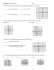### CC Math 8 Name 1.6-1.7 Review Worksheet Date Per ______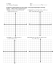### Review for Chapter 8 Test Transformations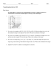### Pyraminx - Beeslife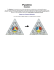### Asia and Europe in Translation Interdisciplinary Perspectives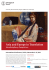### In the punalu`u lo`i, there is a taro patch that is a Rectangle. We need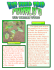### Algebra: Simplifying Algebraic Expressions, Expanding Brackets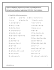### Call for Entries: Translation Internship Experience 2014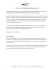### daniel f. gerhartz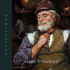### HSM12CC_GM_06_08_CM